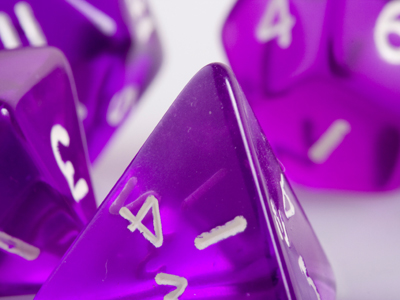Find out about probability in this math quiz.

# Probability

This Math quiz is called 'Probability' and it has been written by teachers to help you if you are studying the subject at middle school. Playing educational quizzes is a fabulous way to learn if you are in the 6th, 7th or 8th grade - aged 11 to 14.

It costs only \$12.50 per month to play this quiz and over 3,500 others that help you with your school work. You can subscribe on the page at Join Us

Probability is all about how likely it is for a specific event to occur. We see it used all around us, in weather forecasts, the lottery, betting on the horses and so on. This middle school Math quiz focuses on the mathematical way of working with probability, which is on a scale from zero (impossible) to 1 (absolutely will happen). The probability of an event occurring is written as a decimal or a fraction, so make sure you can work with both!

To calculate the probability of an event, we need to know the number of successful outcomes (e.g. the number of red counters in a bag) and divide it by the total number of outcomes (e.g. the total number of counters in a bag). The result will always be between 0 and 1 – if not, check your working!

For a single event, it either happens or it doesn’t – so the probability of it happening, P(happens) added to the probability of it not happening, P(not happens) will always equal 1.

Things start to get interesting when there are two events. Events are INDEPENDENT if the outcome of one does not affect the outcome of the other – if I toss a coin and roll a dice, the result of the coin toss will have no bearing on the dice roll. If I take a card from a pack of playing cards, and don’t replace it, then the outcome of the second card drawn is affected by the first card, and so these two events are not independent.

Two events are MUTUALLY EXCLUSIVE if they cannot happen at the same time. In a coin toss, I can’t get both a head and a tail in one toss, so these events are mutually exclusive.

Question 1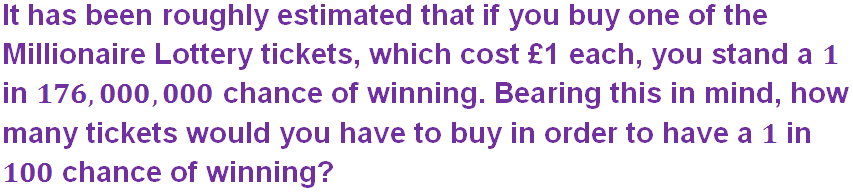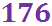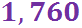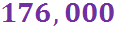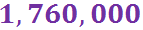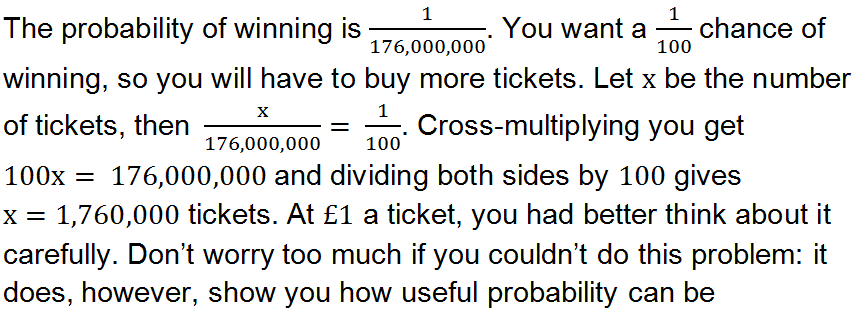Question 2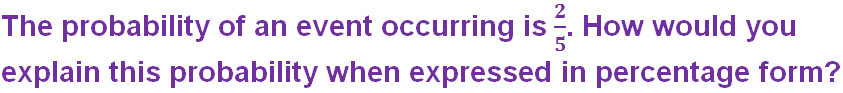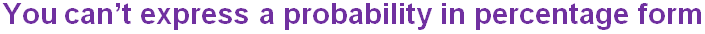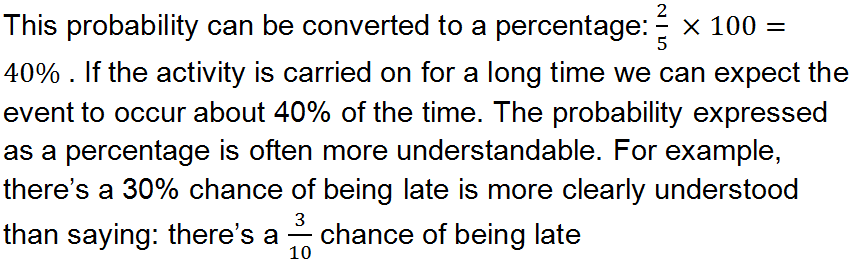Question 3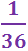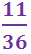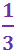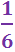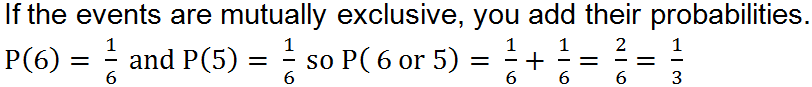Question 4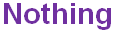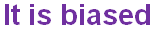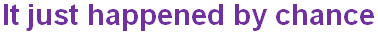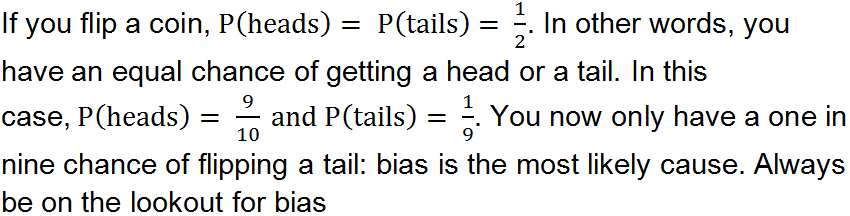Question 5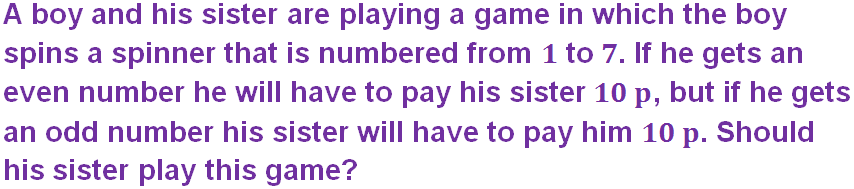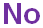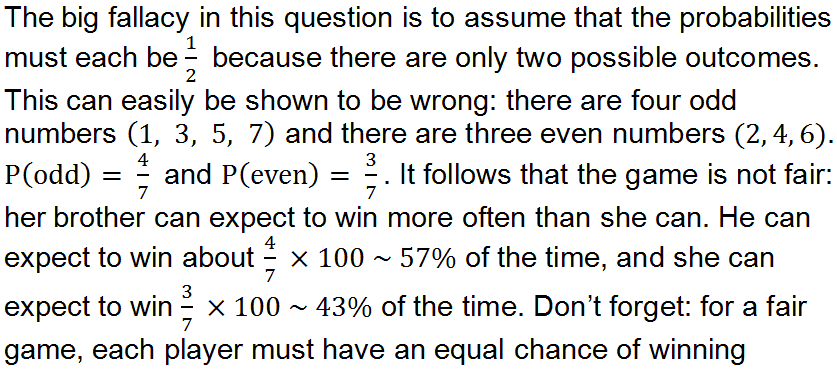Question 6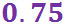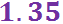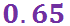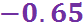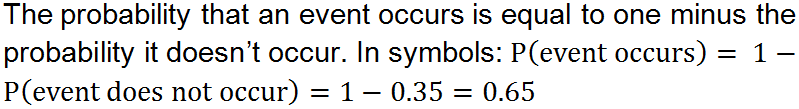Question 7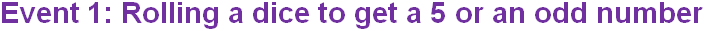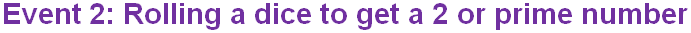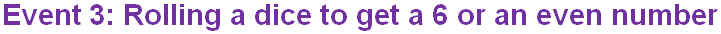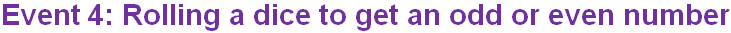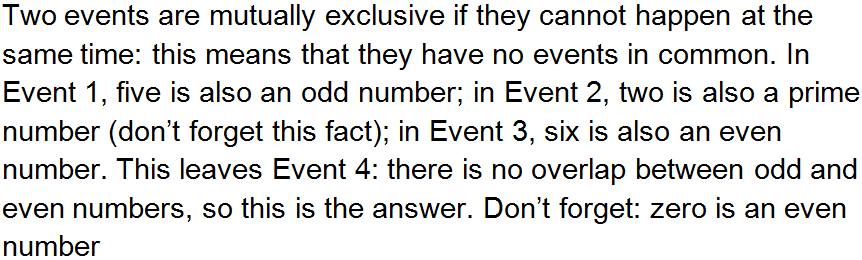Question 8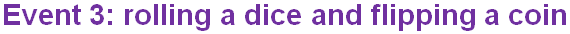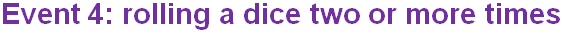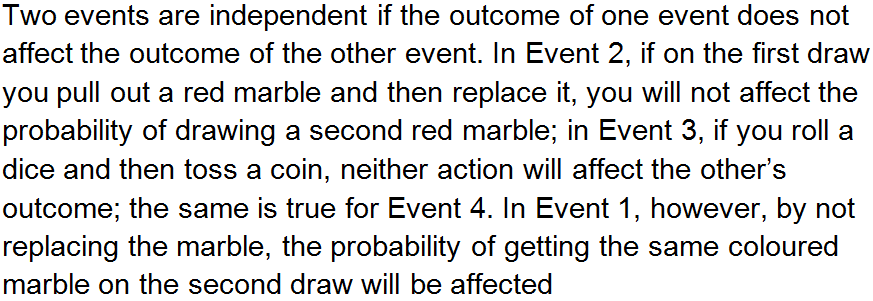Question 9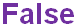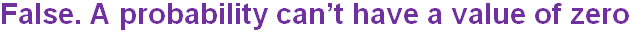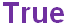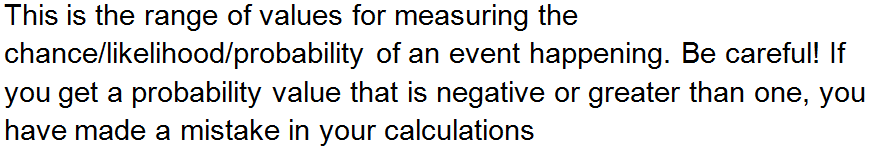Question 10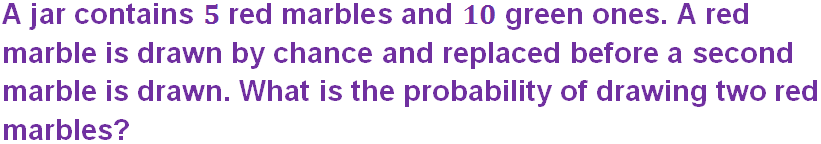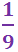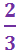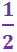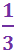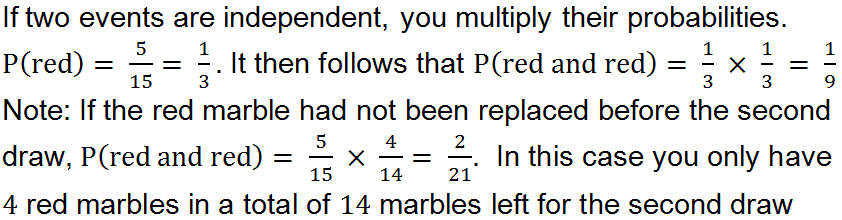Author:  Frank Evans